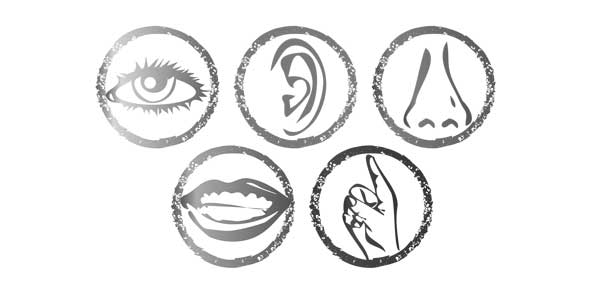# Review

7 QuestionsSettingsRelated Topics
• 1.
1. What is the median age in months for a student in this 6th grade class?  Explain how you determined your answer.
• 2.
1. How many trees are represented in the histogram?
• 3.
What is the mean
• 4.
What is the median
• 5.
What is the mode
• 6.
Compare the number of students between 132.5 and 139 months and the number of students between 139 and 142.5 months.
• A.

The same

• B.

More students n the 132 and 139 range

• C.

More students in the 139 and 142 range

• D.

More girls then boys

• E.

More boys them girls

• 7.
How do the values of the mean, median and mode compare?
• A.

All different number

• B.

They are all around the number 3

• C.

They are all even numbers

• D.

They have nothing in common